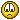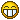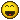## Scaling lower third graphics

Moderator: SecondMan

joergmark
Posts: 46
Joined: Sat Jan 03, 2015 1:59 am
Location: Marxzell, Germany
Contact:

### Scaling lower third graphics

Hi, I would like to create some lower third animations which scale with the text length used...

e.g. if I have a short name and a long one in there the animation adapts to the text.

...any ideas how to achieve this?

cheers
Jörg-Mark

SirEdric
Fusionator
Posts: 1560
Joined: Tue Aug 05, 2014 10:04 am
Been thanked: 45 times
Contact:

### Re: Scaling lower third graphics

What are you animating?
In case you use a follower, there are delay options for "between each character", and "between first and last character".
Where "between each character" would adapt to the length of the text.

joergmark
Posts: 46
Joined: Sat Jan 03, 2015 1:59 am
Location: Marxzell, Germany
Contact:

### Re: Scaling lower third graphics

I think of animations like these:

https://www.rocketstock.com/free-after- ... -premiere/

SirEdric
Fusionator
Posts: 1560
Joined: Tue Aug 05, 2014 10:04 am
Been thanked: 45 times
Contact:

### Re: Scaling lower third graphics

Right....so it's not individual characters, but rather sliding entire lines of text...hmmmm....

Miltos
Fusionista
Posts: 434
Joined: Wed Aug 06, 2014 2:18 am
Contact:

### Re: Scaling lower third graphics

Those don't look like they need to have their animation lengths adjusted according to the size of the names.

My mind initially went to what Eric said as well but the video you posted looks like standard lower third stuff. If anything might need adjustment perhaps it would be a background panel size under a name or something.

joergmark
Posts: 46
Joined: Sat Jan 03, 2015 1:59 am
Location: Marxzell, Germany
Contact:

### Re: Scaling lower third graphics

yes, exactly - or en element which slides to the end of the underlay background... and has to stop at the correct end of the text

SirEdric
Fusionator
Posts: 1560
Joined: Tue Aug 05, 2014 10:04 am
Been thanked: 45 times
Contact:

### Re: Scaling lower third graphics

Ahh...okay... so it's not about the *speed* of the text, but size and positioning of graphical elements.
One very easy way to to that is utilize Text+'s shading tabs.
Each element can be animated separately, plus it scales with the text...Code: Select all

{
Tools = ordered() {
Text2 = TextPlus {
CtrlWZoom = false,
Inputs = {
Width = Input { Value = 1920, },
Height = Input { Value = 1080, },
["Gamut.SLogVersion"] = Input { Value = FuID { "SLog2" }, },
Font = Input { Value = "Open Sans", },
StyledText = Input { Value = "Some Name", },
Style = Input { Value = "Bold", },
ManualFontKerningPlacement = Input {
Value = StyledText {
Array = {
},
Value = ""
},
},
SelectElement = Input { Value = 1, },
Select = Input { Value = 1, },
Enabled2 = Input { Value = 1, },
ElementShape2 = Input { Value = 2, },
Level2 = Input { Value = 1, },
Red2 = Input { Value = 0, },
Green2 = Input { Value = 0.666666666666667, },
Blue2 = Input { Value = 1, },
Transform2 = Input { Value = 1, },
},
ViewInfo = OperatorInfo { Pos = { -165, -49.5 } },
}
},
ActiveTool = "Text2"
}

Dunn
Moderator
Posts: 474
Joined: Mon Aug 04, 2014 4:27 am
Location: Hamburg, Germany
Been thanked: 2 times
Contact:

### Re: Scaling lower third graphics

Well, not sure how you would do it in 2d .. but if you want a 3d proto, you can use the UV tool to get the size of the text.
The *Only* problem with this setup is that you have to Update the UV tool manually ( @SirEdric ,.. I still haven't found away to do this by code )

Edit : nice one SirEdric !

SirEdric
Fusionator
Posts: 1560
Joined: Tue Aug 05, 2014 10:04 am
Been thanked: 45 times
Contact:

### Re: Scaling lower third graphics

The UVMap still crashes Fusion when "Fit" is triggered from Script...In 2D it's indeed rather simple...Code: Select all

{
Tools = ordered() {
AutoDomain1 = AutoDomain {
Inputs = {
Input = Input {
SourceOp = "Text1",
Source = "Output",
},
},
ViewInfo = OperatorInfo { Pos = { 0, 16.5 } },
},
Crop2 = Crop {
Inputs = {
XOffset = Input {
Value = 501,
Expression = "self.Input.DataWindow",
},
YOffset = Input {
Value = 486,
Expression = "self.Input.DataWindow",
},
XSize = Input {
Value = 919,
Expression = "self.Input.DataWindow-self.Input.DataWindow",
},
YSize = Input {
Value = 91,
Expression = "self.Input.DataWindow-self.Input.DataWindow",
},
Input = Input {
SourceOp = "AutoDomain1",
Source = "Output",
},
},
ViewInfo = OperatorInfo { Pos = { 110, 16.5 } },
},
Text1 = TextPlus {
Inputs = {
Width = Input { Value = 1920, },
Height = Input { Value = 1080, },
["Gamut.SLogVersion"] = Input { Value = FuID { "SLog2" }, },
Font = Input { Value = "Open Sans", },
StyledText = Input { Value = "One Cool Guy is here", },
Style = Input { Value = "Bold", },
ManualFontKerningPlacement = Input {
Value = StyledText {
Array = {
},
Value = ""
},
},
Alignment = Input { Value = 1, },
},
ViewInfo = OperatorInfo { Pos = { -165, 16.5 } },
}
}
}

Dunn
Moderator
Posts: 474
Joined: Mon Aug 04, 2014 4:27 am
Location: Hamburg, Germany
Been thanked: 2 times
Contact:

### Re: Scaling lower third graphics

One thing I noticed your 3d text has to have an Extrusion Depth ( something like 0.0001 will do )
hopefully this will be fixed at some point of time in the future. The future to comeSirEdric
Fusionator
Posts: 1560
Joined: Tue Aug 05, 2014 10:04 am
Been thanked: 45 times
Contact:

### Re: Scaling lower third graphics

Actually....using the 2D crop method one could even get a bit more fancy...Code: Select all

{
Tools = ordered() {
Polygon2 = PolylineMask {
DrawMode = "InsertAndModify",
DrawMode2 = "InsertAndModify",
Inputs = {
Invert = Input { Value = 1, },
MaskWidth = Input { Value = 1920, },
MaskHeight = Input { Value = 1080, },
PixelAspect = Input { Value = { 1, 1 }, },
ClippingMode = Input { Value = FuID { "None" }, },
Polyline = Input {
SourceOp = "Polygon2Polyline",
Source = "Value",
},
Polyline2 = Input {
Value = Polyline {
},
Disabled = true,
},
},
ViewInfo = OperatorInfo { Pos = { -220, -49.5 } },
},
Polygon2Polyline = BezierSpline {
SplineColor = { Red = 173, Green = 255, Blue = 47 },
NameSet = true,
KeyFrames = {
 = { 0, Flags = { Linear = true, LockedY = true }, Value = Polyline {
Closed = true,
Points = {
{ Linear = true, X = 0.379759516051872, Y = 0.626760560442025, LX = 0.0641282512971219, LY = -0.0140845041039964, RX = 0.0501001918403483, RY = -0.474178403056284 },
{ Linear = true, X = 0.530060091572916, Y = -0.795774648726826, LX = -0.0501001918403483, LY = 0.474178403056284, RX = 0.0140280594567737, RY = 0.460093898952287 },
{ Linear = true, X = 0.572144269943237, Y = 0.584507048130035, LX = -0.0140280594567737, LY = -0.460093898952287, RX = -0.0641282512971219, RY = 0.0140845041039964 }
}
} }
}
},
Background3 = Background {
Inputs = {
Width = Input {
Value = 1653,
Expression = "Crop2.Output.OriginalWidth+ExtendX",
},
Height = Input {
Value = 116,
Expression = "Crop2.Output.OriginalHeight+ExtendY",
},
["Gamut.SLogVersion"] = Input { Value = FuID { "SLog2" }, },
TopLeftGreen = Input { Value = 0.66650390625, },
TopLeftBlue = Input { Value = 1, },
ExtendX = Input { Value = 200, },
ExtendY = Input { Value = 25, },
EffectMask = Input {
SourceOp = "Polygon2",
}
},
ViewInfo = OperatorInfo { Pos = { -110, -49.5 } },
UserControls = ordered() {
ExtendX = {
LINKS_Name = "Extend X",
INPID_InputControl = "SliderControl",
INP_Default = 0,
INP_Integer = true,
INP_MinScale = 0,
INP_MaxScale = 100,
INP_MinAllowed = -1000000,
INP_MaxAllowed = 1000000,
ICS_ControlPage = "Image"
},
ExtendY = {
LINKS_Name = "Extend Y",
INPID_InputControl = "SliderControl",
INP_Default = 0,
INP_Integer = true,
INP_MinScale = 0,
INP_MaxScale = 100,
ICS_ControlPage = "Image"
}
}
},
Crop2 = Crop {
Inputs = {
XOffset = Input {
Value = 223,
Expression = "self.Input.DataWindow",
},
YOffset = Input {
Value = 486,
Expression = "self.Input.DataWindow",
},
XSize = Input {
Value = 1453,
Expression = "self.Input.DataWindow-self.Input.DataWindow",
},
YSize = Input {
Value = 91,
Expression = "self.Input.DataWindow-self.Input.DataWindow",
},
Input = Input {
SourceOp = "AutoDomain1",
Source = "Output",
},
},
ViewInfo = OperatorInfo { Pos = { -110, 16.5 } },
},
Text1 = TextPlus {
Inputs = {
Width = Input { Value = 1920, },
Height = Input { Value = 1080, },
["Gamut.SLogVersion"] = Input { Value = FuID { "SLog2" }, },
Font = Input { Value = "Open Sans", },
StyledText = Input { Value = "One Cool Guy is here talking shit ", },
Style = Input { Value = "Bold", },
ManualFontKerningPlacement = Input {
Value = StyledText {
Array = {
},
Value = ""
},
},
Alignment = Input { Value = 1, },
},
ViewInfo = OperatorInfo { Pos = { -330, 16.5 } },
},
AutoDomain1 = AutoDomain {
Inputs = {
Input = Input {
SourceOp = "Text1",
Source = "Output",
},
},
ViewInfo = OperatorInfo { Pos = { -220, 16.5 } },
},
tMove = Transform {
CtrlWZoom = false,
NameSet = true,
Inputs = {
Center = Input {
SourceOp = "XYPath1",
Source = "Value",
},
Input = Input {
SourceOp = "Crop2",
Source = "Output",
},
mSpeed = Input { Value = 10, },
},
ViewInfo = OperatorInfo { Pos = { 0, 16.5 } },
UserControls = ordered() {
mSpeed = {
INPID_InputControl = "SliderControl",
INP_Default = 1,
INP_Integer = false,
INP_MinScale = 0.100000001490116,
INP_MaxScale = 10,
ICS_ControlPage = "Controls",
INP_External = false
}
}
},
XYPath1 = XYPath {
ShowKeyPoints = false,
DrawMode = "ModifyOnly",
Inputs = {
X = Input {
SourceOp = "XYPath1X",
Source = "Value",
},
Y = Input {
SourceOp = "XYPath1Y",
Source = "Value",
},
},
},
XYPath1X = BezierSpline {
SplineColor = { Red = 255, Green = 0, Blue = 0 },
NameSet = true,
KeyFrames = {
 = { -0.570441280946771, RH = { 8.90382750665286, 1.56647945757065 }, Flags = { Linear = true } },
 = { 0.5, LH = { 16.666675, 0.5 } }
}
},
XYPath1Y = BezierSpline {
SplineColor = { Red = 0, Green = 255, Blue = 0 },
NameSet = true,
KeyFrames = {
 = { 0.5, RH = { 8.33333333333333, 0.5 }, Flags = { Linear = true } },
 = { 0.5, LH = { 16.6666666666667, 0.5 }, Flags = { Linear = true } }
}
},
Transform1 = Transform {
Inputs = {
Center = Input {
SourceOp = "XYPath2",
Source = "Value",
},
Input = Input {
SourceOp = "Background3",
Source = "Output",
},
},
ViewInfo = OperatorInfo { Pos = { 0, -49.5 } },
},
XYPath2 = XYPath {
ShowKeyPoints = false,
DrawMode = "ModifyOnly",
Inputs = {
X = Input {
SourceOp = "XYPath2X",
Source = "Value",
},
Y = Input {
SourceOp = "XYPath2Y",
Source = "Value",
},
},
},
XYPath2X = BezierSpline {
SplineColor = { Red = 255, Green = 0, Blue = 0 },
NameSet = true,
KeyFrames = {
 = { 1.50560224089636, RH = { 8.333325, -0.505602240896359 }, Flags = { Linear = true } },
 = { 0.5, LH = { 16.666675, 0.5 } }
}
},
XYPath2Y = BezierSpline {
SplineColor = { Red = 0, Green = 255, Blue = 0 },
NameSet = true,
KeyFrames = {
 = { 0.5, RH = { 8.33333333333333, 0.5 }, Flags = { Linear = true } },
 = { 0.5, LH = { 16.6666666666667, 0.5 }, Flags = { Linear = true } }
}
},
Merge3 = Merge {
Inputs = {
Background = Input {
SourceOp = "Transform1",
Source = "Output",
},
Foreground = Input {
SourceOp = "tMove",
Source = "Output",
},
Center = Input { Value = { 0.457832618025751, 0.5 }, },
PerformDepthMerge = Input { Value = 0, },
},
ViewInfo = OperatorInfo { Pos = { 110, 16.5 } },
}
}
}

SecondMan
Posts: 3102
Joined: Thu Jul 31, 2014 5:31 pm
Been thanked: 23 times
Contact:

### Re: Scaling lower third graphics

I read that as "one cool guy taking a"

SirEdric
Fusionator
Posts: 1560
Joined: Tue Aug 05, 2014 10:04 am
Been thanked: 45 times
Contact:

### Re: Scaling lower third graphics

Damn...should have learned by now to not use fekal dummy texts....joergmark
Posts: 46
Joined: Sat Jan 03, 2015 1:59 am
Location: Marxzell, Germany
Contact:

### Re: Scaling lower third graphics

WOW - Thank YouIll try what I can do with this...